# College Physics : Pressure

## Example Questions

### Example Question #1 : Fluids

Consider the image shown below.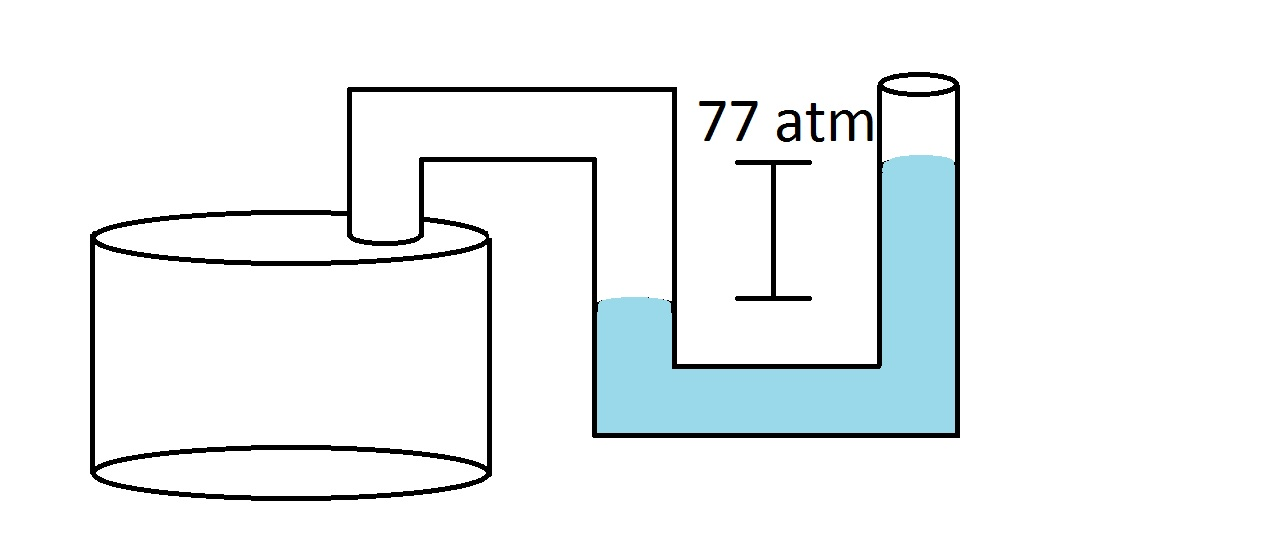In the container on the left, there is a gas at an unknown pressure. The tubing connected to the container is open to the atmosphere, which has a pressure of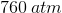. If the liquid contained within the tubing shows a pressure difference of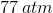, as in the above image, what is the pressure of the gas in the container?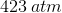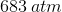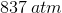Explanation:

In this question, we're told that an unknown gas is held within a container. Connected to this container is a tube open to the atmosphere which also contains a liquid. Based on the image shown, the height difference of the liquid levels, and the atmospheric pressure, we're asked to determine the pressure of the unknown gas inside the container.

This is a fairly straight-forward problem that asks us about pressure. Because of the way the tubing is situated, the pressure from the atmosphere will push down on the right side of the tubing, while the pressure from the unknown gas in the container will push down on the left part of the tubing. Since the liquid level on the left is lower than on the right, we can infer that the gas in the container is "pushing" on the liquid more strongly than the atmosphere on the other side. Consequently, we know that the gas in the container has a pressure greater than atmospheric pressure.

The difference in height of the liquid levels allows us to quantitatively determine how much greater this pressure is. Since the height difference shows up as, we know that the gas in the container ismore than atmospheric pressure.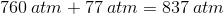### Example Question #1 : Fluids

An object is initially submerged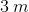below the surface of water. If this same object is then lowered to a depth of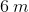below the surface of water, how does the absolute pressure on the object change?

The absolute pressure slightly decreases

The absolute pressure doubles

The absolute pressure slightly increases

The absolute pressure halves

The absolute pressure slightly increases

Explanation:

For this question, we need to understand the relationship between gauge pressure, ambient pressure, and absolute pressure.

The absolute pressure that an object experiences has two components; ambient and gauge pressure. The ambient pressure is an arbitrarily defined reference pressure that acts on the object. Generally, atmospheric pressure at sea level is used as the reference value, which is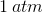. The gauge pressure is measured relative to the ambient pressure that has been used as a reference point. Gauge pressure is dependent on the density of the fluid in which the object is submerged together with the distance under which the object is submerged in the fluid.

The equation for absolute pressure can be expressed as follows.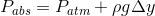In the above expression,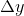is one component of the gauge pressure and refers to the distance of the object from the surface of water. As the object is submerged deeper, it will experience a greater gauge pressure and thus a greater absolute pressure.

But what happens ifwere doubled fromto, as stated in the question stem? This would certainly cause the gauge pressure to double, but would it cause the absolute pressure to double? The answer is no; there is still the atmospheric pressure to take into account, which maintains the same value in both cases. Because we are increasing the gauge pressure, we know that absolute pressure will increase, but not to the extent of doubling. Thus, we know that it will increase slightly.

### All College Physics Resources